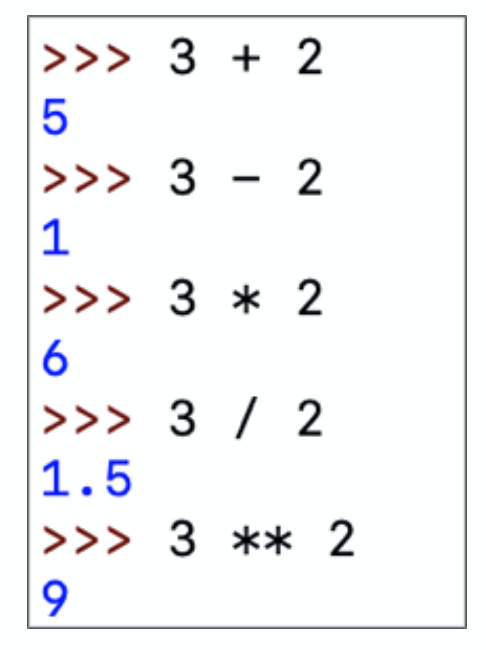Search
• Kaitlyn Flowers

# Python Class, Week 2!

Here's the review of our second class. The class powerpoint is linked below:

Week 2 Python Class
.pdf
PDF • 113KB

## Some more Basics:

In Python we use these math operators for simple equations.Subtraction: -

Multiplication: *

Division: /

Exponents **

We also use these comparison Operators to compare different objects:

Equal: ==

Not Equal: !=

Greater than/lesser than: < or >

Greater/Lesser than or equal to: <= or >=

Here are Python's basic data types:Integer/whole number: int

String (text): str

Float (decimal number): float

Boolean (true/false): bool

## Lists:

• A list is a data structure that allows us to group data together and assign them to a variable.

• In other programming languages such as Java, a list is known as an array

• We use square brackets ('[' and ']') to define a list, and separate the elements or items in our list using commas.

`names = ["Rachael", "Mana", "Minseo"]`

We can enter any type of data in our list:

`example_list = [42, "hello, world!", 3.0, True]`

...and we can print a list like this:

```print(names)
print(example_list)```

Accessing Items in a List:

- lists use an index to refer to a specific position of time

- Index starts at 0, so the index of the last item will be 1 less than the total number of items in your list

- To access an element of a list using index, we write: list[index]

```names #This should print 'Minseo'
example_list #This should print 42```

Looping through a List:

Programmers often use for-loops and while loops with lists. You can iterate (go over the range of items in a list and perform the same task) through the list using these loops. Let's take a look at an example:

```scores = [96, 93, 84, 90, 98, 81]
print("Here are your scores for the term:")
for score in scores:
print(score)```

For Practice... go to https://codingbat.com/python. There you can practice basic syntax and lists!

If you need anything, don't hesitate to reach out!

Mana Vale: nvale@exeter.edu

Rachael Kim: skim1@exeter.edu

Kaitlyn Flowers: kflowers@exeter.edu

Celine Tan: cltan@exeter.edu

Joey Dong: jdong1@exeter.edu

Molly Pate: mpate@exeter.edu

See All